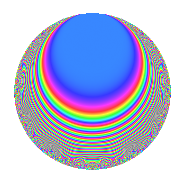# Properties

 Label 126.2.tLevel 126 Weight 2 Character orbit t Rep. character $$\chi_{126}(47,\cdot)$$ Character field $$\Q(\zeta_{6})$$ Dimension 16 Newforms 1 Sturm bound 48 Trace bound 0

# Learn more about

## Defining parameters

 Level: $$N$$ = $$126 = 2 \cdot 3^{2} \cdot 7$$ Weight: $$k$$ = $$2$$ Character orbit: $$[\chi]$$ = 126.t (of order $$6$$ and degree $$2$$) Character conductor: $$\operatorname{cond}(\chi)$$ = $$63$$ Character field: $$\Q(\zeta_{6})$$ Newforms: $$1$$ Sturm bound: $$48$$ Trace bound: $$0$$

## Dimensions

The following table gives the dimensions of various subspaces of $$M_{2}(126, [\chi])$$.

Total New Old
Modular forms 56 16 40
Cusp forms 40 16 24
Eisenstein series 16 0 16

## Trace form

 $$16q$$ $$\mathstrut +\mathstrut 8q^{4}$$ $$\mathstrut +\mathstrut 2q^{7}$$ $$\mathstrut -\mathstrut 6q^{9}$$ $$\mathstrut +\mathstrut O(q^{10})$$ $$16q$$ $$\mathstrut +\mathstrut 8q^{4}$$ $$\mathstrut +\mathstrut 2q^{7}$$ $$\mathstrut -\mathstrut 6q^{9}$$ $$\mathstrut -\mathstrut 6q^{13}$$ $$\mathstrut -\mathstrut 6q^{14}$$ $$\mathstrut -\mathstrut 18q^{15}$$ $$\mathstrut -\mathstrut 8q^{16}$$ $$\mathstrut +\mathstrut 18q^{17}$$ $$\mathstrut +\mathstrut 12q^{18}$$ $$\mathstrut -\mathstrut 18q^{21}$$ $$\mathstrut -\mathstrut 6q^{24}$$ $$\mathstrut +\mathstrut 16q^{25}$$ $$\mathstrut -\mathstrut 12q^{26}$$ $$\mathstrut -\mathstrut 36q^{27}$$ $$\mathstrut -\mathstrut 2q^{28}$$ $$\mathstrut +\mathstrut 6q^{29}$$ $$\mathstrut -\mathstrut 18q^{30}$$ $$\mathstrut +\mathstrut 6q^{31}$$ $$\mathstrut +\mathstrut 18q^{33}$$ $$\mathstrut -\mathstrut 30q^{35}$$ $$\mathstrut -\mathstrut 2q^{37}$$ $$\mathstrut -\mathstrut 30q^{39}$$ $$\mathstrut +\mathstrut 6q^{41}$$ $$\mathstrut +\mathstrut 30q^{42}$$ $$\mathstrut -\mathstrut 2q^{43}$$ $$\mathstrut +\mathstrut 12q^{44}$$ $$\mathstrut +\mathstrut 12q^{45}$$ $$\mathstrut +\mathstrut 6q^{46}$$ $$\mathstrut -\mathstrut 18q^{47}$$ $$\mathstrut +\mathstrut 10q^{49}$$ $$\mathstrut -\mathstrut 12q^{50}$$ $$\mathstrut +\mathstrut 36q^{53}$$ $$\mathstrut +\mathstrut 18q^{54}$$ $$\mathstrut +\mathstrut 6q^{57}$$ $$\mathstrut -\mathstrut 12q^{58}$$ $$\mathstrut +\mathstrut 30q^{59}$$ $$\mathstrut -\mathstrut 6q^{60}$$ $$\mathstrut -\mathstrut 60q^{61}$$ $$\mathstrut -\mathstrut 36q^{62}$$ $$\mathstrut +\mathstrut 42q^{63}$$ $$\mathstrut -\mathstrut 16q^{64}$$ $$\mathstrut +\mathstrut 42q^{65}$$ $$\mathstrut +\mathstrut 48q^{66}$$ $$\mathstrut +\mathstrut 14q^{67}$$ $$\mathstrut +\mathstrut 36q^{68}$$ $$\mathstrut +\mathstrut 42q^{69}$$ $$\mathstrut +\mathstrut 30q^{75}$$ $$\mathstrut -\mathstrut 18q^{77}$$ $$\mathstrut -\mathstrut 16q^{79}$$ $$\mathstrut +\mathstrut 54q^{81}$$ $$\mathstrut -\mathstrut 18q^{84}$$ $$\mathstrut -\mathstrut 12q^{85}$$ $$\mathstrut -\mathstrut 48q^{87}$$ $$\mathstrut +\mathstrut 24q^{89}$$ $$\mathstrut -\mathstrut 18q^{90}$$ $$\mathstrut -\mathstrut 12q^{91}$$ $$\mathstrut +\mathstrut 6q^{92}$$ $$\mathstrut +\mathstrut 30q^{93}$$ $$\mathstrut -\mathstrut 66q^{95}$$ $$\mathstrut -\mathstrut 6q^{96}$$ $$\mathstrut -\mathstrut 6q^{97}$$ $$\mathstrut +\mathstrut 24q^{98}$$ $$\mathstrut +\mathstrut 18q^{99}$$ $$\mathstrut +\mathstrut O(q^{100})$$

## Decomposition of $$S_{2}^{\mathrm{new}}(126, [\chi])$$ into irreducible Hecke orbits

Label Dim. $$A$$ Field CM Traces $q$-expansion
$$a_2$$ $$a_3$$ $$a_5$$ $$a_7$$
126.2.t.a $$16$$ $$1.006$$ $$\mathbb{Q}[x]/(x^{16} - \cdots)$$ None $$0$$ $$0$$ $$0$$ $$2$$ $$q+(-\beta _{1}+\beta _{6})q^{2}+(-\beta _{11}-\beta _{14})q^{3}+\cdots$$

## Decomposition of $$S_{2}^{\mathrm{old}}(126, [\chi])$$ into lower level spaces

$$S_{2}^{\mathrm{old}}(126, [\chi]) \cong$$ $$S_{2}^{\mathrm{new}}(63, [\chi])$$$$^{\oplus 2}$$